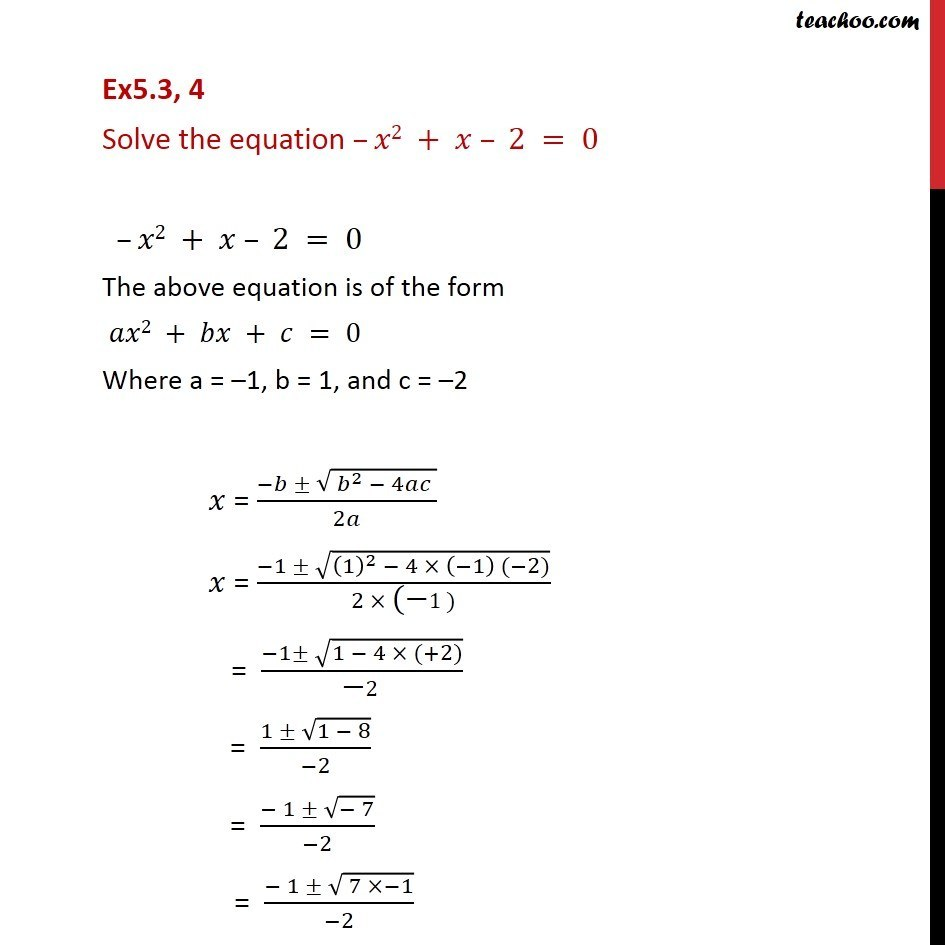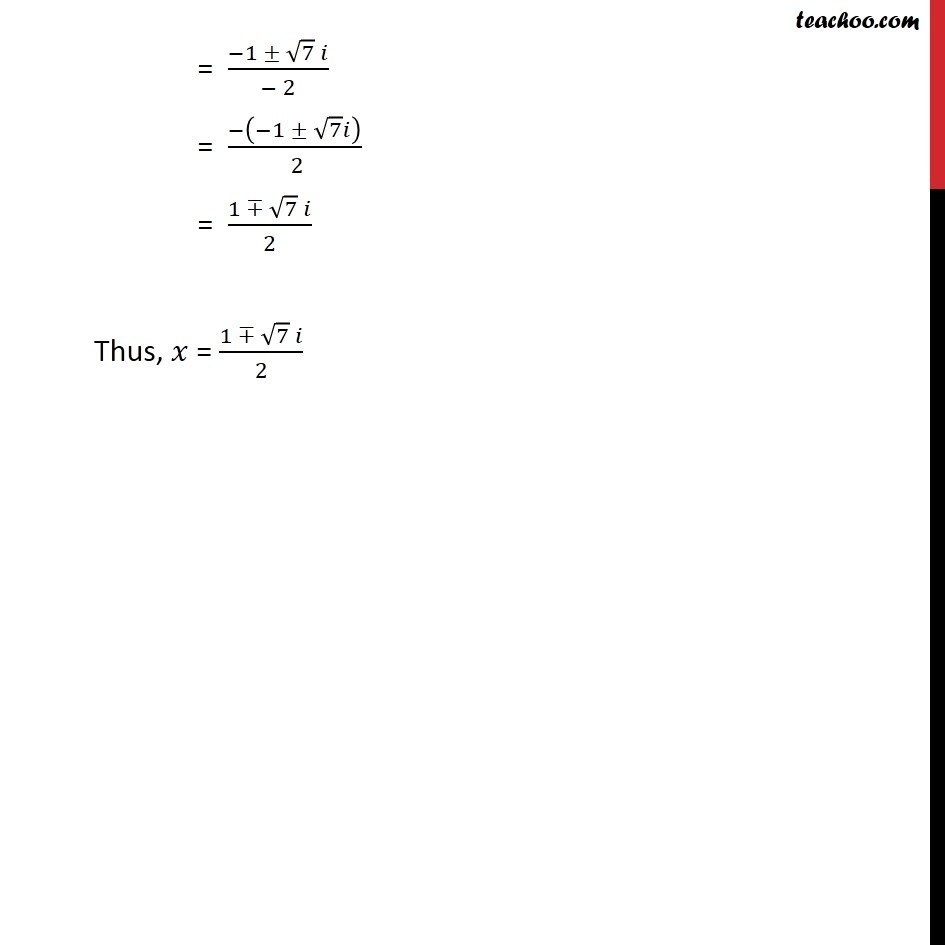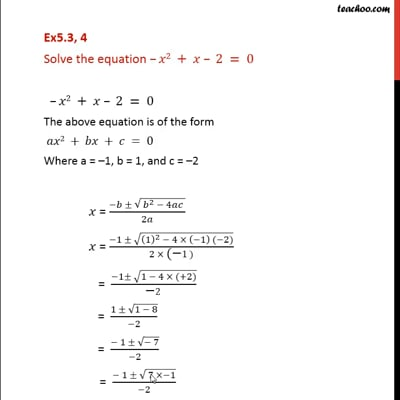Ex 5.3

Chapter 5 Class 11 Complex Numbers
Serial order wiseThis video is only available for Teachoo black users

Solve all your doubts with Teachoo Black (new monthly pack available now!)

### Transcript

Ex5.3, 4 Solve the equation –𝑥2 + 𝑥 – 2 = 0 –𝑥2 + 𝑥 – 2 = 0 The above equation is of the form 𝑎𝑥2 + 𝑏𝑥 + 𝑐 = 0 Where a = –1, b = 1, and c = –2 𝑥 = (−𝑏 ± √( 𝑏^2 − 4𝑎𝑐 ))/2𝑎 𝑥 = (−1 ± √((1)^2 − 4 × (−1) (−2)))/(2 × "(−" 1)) = (−1± √(1 − 4 × (+2)))/("−" 2) = (1 ± √(1 − 8))/(−2) = (− 1 ± √(− 7))/(−2) = (− 1 ± √( 7 ×−1))/(−2) = (−1 ± √7 𝑖)/(− 2) = (−(−1 ± √7 𝑖))/( 2) = (1 ∓ √7 𝑖)/2 Thus, 𝑥 = (1 ∓ √7 𝑖)/2